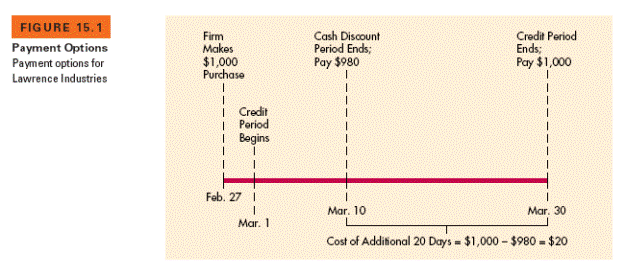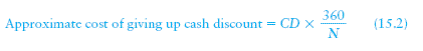# in the preceding example we saw that lawrence industries could take the cash discoun 681671

In the preceding example, we saw that Lawrence Industries could take the cash discount on its February 27 purchase by paying \$980 on March 10. If Lawrence gives up the cash discount, payment can be made on March 30. To keep its money for an extra 20 days, the firm must give up an opportunity to pay \$980 for its \$1,000 purchase. In other words, it will cost the firm \$20 to delay payment for 20 days. Figure 15.1 shows the payment options that are open to the company. To calculate the cost of giving up the cash discount, the true purchase price must be viewed as the discounted cost of the merchandise, which is \$980 forLawrence Industries. The annual percentage cost of giving up the cash discount can be calculated using Equation 15.1:1

where

CD = stated cash discount in percentage terms

= number of days that payment can be delayed by giving up the cash discount

Substituting the values for CD (2%) and (20 days) into Equation 15.1 results in an annualized cost of giving up the cash discount of 36.73% [(2% ÷ 98%) x (360 ÷ 20)]. A 360 day year is assumed.2 A simple way toapproximate the cost of giving up a cash discount is to use the stated cash discount percentage, CD, in place of the first term of Equation 15.1:The smaller the cash discount, the closer the approximation to the actual cost of giving it up. Using this approximation, the cost of giving up the cash discount for Lawrence Industries is 36% [2% x (360 ÷ 20)].